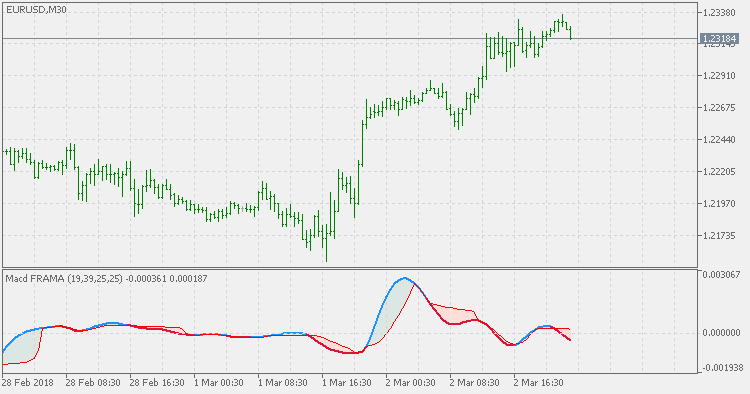Interesting script?
So post a link to it -
let others appraise it

You liked the script? Try it in the MetaTrader 5 terminalViews:
3178
Rating:
Published:
2018.03.05 13:53
Updated:
2018.03.06 16:27

Fractal Adaptive Moving Average Technical Indicator (FRAMA) was developed by John Ehlers. This indicator is constructed based on the algorithm of the Exponential Moving Average, in which the smoothing factor is calculated based on the current fractal dimension of the price series. The advantage of FRAMA is the possibility to follow strong trend movements and to sufficiently slow down at the moments of price consolidation.

Using Fractal Adaptive Moving Average (FRAMA) for MACD calculation is somewhat difficult if some additional steps are not done. In this indicator an addition of smoothing is added to make the Fractal Adaptive MACD more to what we expect from MACD type indicators.

Without smoothing it is "nervous" (too many signals) which is due to its adaptive nature (too fast a reaction when we expect a lag - which is the essential part of any MACD indicator - the "convergence/divergence" part of MACD can happen only if there is some reasonable lag). Super smoother is used in this case for smoothing (there might be some other choices too, but since we needed smoother result and minimal lag addition caused by smoothing, super smoother is a reasonable choice).Signal line in this indicator is a FRAMA too, which makes it an "all FRAMA MACD" indicator.Instantaneous Trend Line bands (generalized)

This version extends the Instantaneous Trend Line indicator with a sort of bands to make it easier to spot trend change and to filter out some false signals that can be produced if only the slope of the ITL indicator is takes for a signal. And it also differs from the original in one important part: instead of using fractional alpha parameter for calculation, this version uses period which makes it the same category as averages are and the usage becomes a bit more intuitive than the original version.Instantaneous Trend Line (generalized)

This version differs from the original Instantaneous Trend Line in one important part: instead of using fractional alpha parameter for calculation, this version uses period which makes it the same category as averages are and the usage becomes a bit more intuitive than the original version.ZigZag separate

ZigZag separate is a MetaTrader 5 version of one indicator that was floating around the net as a wonder indicator for MetaTrader 4.Chande's DMI (Dynamic Momentum Index)

The Dynamic Momentum Index (DMI) is a variable term RSI. When default values are used, the RSI term varies from 3 to 30. The variable time period makes the RSI more responsive to short-term moves. The more volatile the price is, the shorter the time period is. It is interpreted in the same way as the RSI, but provides signals earlier.